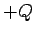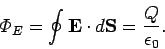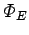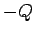Next: Breakdown Up: Capacitance Previous: Capacitance

## Charge Storage

Consider a hollow metal sphere mounted on an insulating stand. The sphere is initially grounded so that no excess charge remains on it. Suppose that we introduce a metal ball, suspended on an insulating thread, through a small hole in the sphere, and then fill in the hole with a metal plug. Let the ball carry a charge. What distribution of charge is induced on the hollow sphere as a result of introducing the positive charge into the cavity?

To answer this question we make use of Gauss' law (see Sect. 4.2)(100)

Assuming that the metal ball is placed at the centre of the hollow sphere, we can use symmetry arguments to deduce that the electric field depends only on the radial distancefrom the centre, and is everywhere directed radially away from the ball. Let us choose a spherical gaussian surface, centred on the ball, which runs through the interior of the hollow metal sphere. We know that the electric field inside a conductor is everywhere zero (see Sect. 4.6), so the electric fluxthrough the surface is also zero. It follows from Gauss' law that zero net charge is enclosed by the surface. Now, there is a chargeon the ball at the centre of the hollow sphere, so there must be an equal and opposite chargedistributed over the interior surface of the sphere (recall that any charge carried on a conductor must reside on its surface). Furthermore, since the sphere is insulated, and was initially uncharged, a chargemust be distributed over its exterior surface. Thus, when the chargeis introduced into the centre of the sphere, there is a redistribution of charge in the sphere such that a positive chargeis repelled to its exterior surface, leaving a negative chargeon the interior surface. (In actuality, free electrons are attracted to the interior surface, exposing positive charges on the exterior surface). Further use of Gauss' law shows that the electric field between the charged ball and the interior surface of the sphere is the same as that generated by a point chargelocated at the centre of the sphere. Likewise, for the electric field exterior to the sphere. The electric field inside the conducting sphere is, of course, zero.

Suppose, finally, that the ball is moved so that it touches the inside of the hollow sphere. The chargeon the interior surface of the sphere cancels the chargeon the ball, leaving the chargedistributed over its exterior surface. Thus, the effect of touching the ball to the inside of the sphere is to transfer the chargefrom the ball to the exterior surface of the sphere. In principle, we can repeat this process, again and again, until a very large amount of charge is accumulated on the outside of the sphere. The idea of transferring charge from one conductor to another by means of internal contact is the theoretical basis of the Van de Graaff generator. In this type of device, charge is continuously transmitted to a conducting sphere by means of a moving belt charged by friction.Next: Breakdown Up: Capacitance Previous: Capacitance
Richard Fitzpatrick 2007-07-14